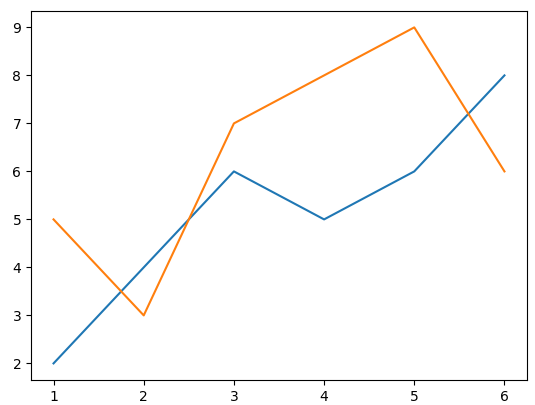# Matplotlib: Draw Multiple Lines with Different Color on the Same Plot

In order to compare values, we can use bar chart in matplotlib. Here is the tutorial:

Matplotlib: Compare Values with Different Color in Bar Chart

However, we also can use multiple line plots to compare. In this tutorial, we will introduce how to draw multiple lines with different color on the same plot.1.Import library

```import matplotlib.pyplot as plt
import numpy as np```

2.Prepare data

```x = [1, 2, 3, 4, 5, 6]
y = [2, 4, 6, 5, 6, 8]
y2 = [5, 3, 7, 8, 9, 6]```

We should notice: x is shared by y and y2.

3.Draw line plot

```fig, ax = plt.subplots()

ax.plot(x, y)
ax.plot(x, y2)
plt.show()```

Run this code, you will see this plot.Moreover, if you want to change the color of each line or add legend, you can do as follows:

```ax.plot(x, y, color = 'green', label = 'Line 1')
ax.plot(x, y2, color = 'red', label = 'Line 2')
ax.legend(loc = 'upper left')
plt.show()```